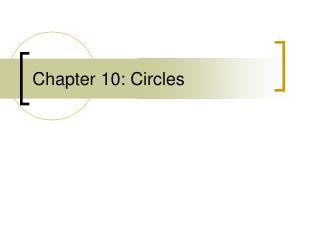DownloadDownload PresentationChapter 10: Circles

# Chapter 10: Circles

Download Presentation## Chapter 10: Circles

- - - - - - - - - - - - - - - - - - - - - - - - - - - E N D - - - - - - - - - - - - - - - - - - - - - - - - - - -
##### Presentation Transcript

1. Chapter 10: Circles

2. 10.1 Circles and Circumference • Name a circle by the letter at the center of the circle • Diameter- segment that extends from one point on the circle to another point on the circle through the center point • Radius- segment that extends from one point on the circle to the center point • Chord- segment that extends from one point on the circle to another point on the circle • Diameter=2 x radius (d=2r) • Circumference: the distance around the circle • C=2πr or C= πd

3. Circle X Diameter- Radius- Chord- A chord B diameter E C X radius D

4. Name the circle • Name the radii • Identify a chord • Identify a diameter

5. Find the circumference of a circle to the nearest hundredth if its radius is 5.3 meters. b. Find the diameter and the radius of a circleto the nearest hundredth if the circumference of the circle is 65.4 feet.

6. 10.2 Angles, Arcs and Chords • 10.2 • Semi-circle: half the circle (180 degrees) • Minor arc: less than 180 degrees • Name with two letters • Major arc: more than 180 degrees • Name with three letters • Minor arc = central angle • Arc length:

7. B Minor arc Minor arc Minor arc = AB or BC Semicircle = ABC or CDA Major arc = ABD or CBD AB + BC = 180 C Central angle X A D Semicircle

8. Find the value of x.

9. Find x and angle AZE

10. 10.3 Arcs and Chords • If two chords are congruent, then their arcs are also congruent • In inscribed quadrilaterals, the opposite angles are supplementary • If a radius or diameter is perpendicular to a chord, it bisects the chord and its arc • If two chords are equidistant from the center of the circle, the chords are congruent

11. A If FE=BC, then arc FE = arc BC Quad. BCEF is an inscribed polygon – opposite angles are supplementary angles B and E & angles F and C Diameter AD is perpendicular to chord EC – so chord EC and arc EC are bisected B F C E D

12. B *You can use the pythagorean theorem to find the radius when a chord is perpendicular to a segment from the center EX = FX so chords AB and CD are congruent because they are equidistant from the center E A X C F D

13. In the circle below, diameter QS is 14 inches long and chord RT is 10 inches long. Find VU.

14. 10.4 Inscribed Angles • Inscribed angle: an angle inside the circle with sides that are chords and a vertex on the edge of the circle • Inscribed angle = ½ intercepted arc • An inscribed right angle, always intercepts a semicircle • If two or more inscribed angles intercept the same arc, they are congruent

15. A Inscribed angles: angle BAC, angle CAD, angle DAE, angle BAD, angle BAE, angle CAE X B Ex: Angle DAE = ½ arc DE C E D

16. A Inscribed angle BAC intercepts a semicircle- so angle BAC =90 Inscribed angles GDF and GEF both intercept arc GF, so the angles are congruent B C D F E G

17. A. Find mX.

18. ALGEBRA Find mR.

19. ALGEBRA Find mI.

20. ALGEBRA Find mB.

21. ALGEBRA Find mD.

22. INSIGNIAS An insignia is an emblem that signifies rank, achievement, membership, and so on. The insignia shown is a quadrilateral inscribed in a circle. Find mS and mT.

23. 10.5 Tangents • Tangent: a line that shares only one point with a circle and is perpendicular to the radius or diameter at that point. • Point of tangency: the point that a tangent shares with a circle • Two lines that are tangent to the same circle and meet at a point, are congruent from that point to the points of tangency

24. Lines AC and AF are tangent to circle X at points B and E respectively -B and E are points of tangency Radius XB is perpendicular to tangent AC at the point of tangency AE and AB are congruent because they are tangent to the same circle from the same point C F E B A

25. A.Copy the figure and draw the common tangents to determine how many there are. If no common tangent exists, choose no common tangent.

26. 10.6 Secants, Tangents, and Angle Measures • Secant and Tangent • Interior angle = ½ intercepted arc • Two Secants: • Interior angle = ½ (sum of intercepted arcs) • Two Secants • Exterior angle = ½ (far arc – close arc) • Two Tangents • Exterior angle = ½ (far arc – close arc)

27. C 2 Secants/chords: Angle 1 = ½ (arc AD + arc CB) Angle 2 = ½ (arc AC + arc DB) B 2 1 A D

28. E Secant ED intersects tangent FC at a point of tangency (point F) Angle 1 = ½ arc FE Angle 2 = arc EA – arc FB 1 F B A 2 D C